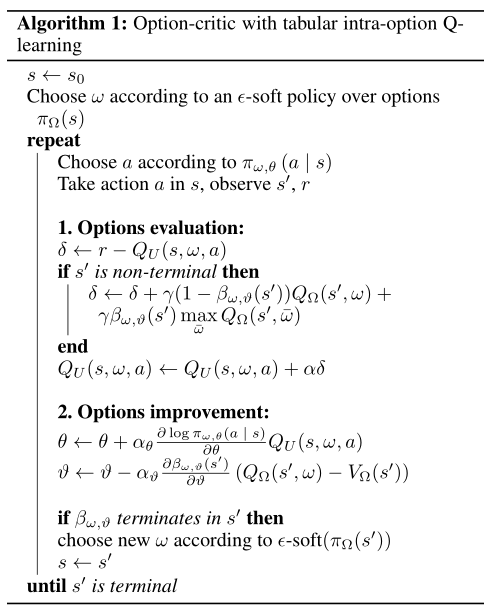MySQL主从分离实现

# Abstract

Temporal abstraction：

# Introduction

How to understand? Option?

1. 我们首先回顾与我们工作的两个主要组成部分相关的背景：policy gradient method 和 option。
2. 然后我们描述了我们方法的核心思想：the intra-option policy 和 termination gradient theorems。附加技术细节见附录。
3. 实验结果表明，我们的方法能够有效地学习有意义的时间扩展行为。与其他方法不同，我们只需要指定所需选项的数量；不需要有子目标、额外奖励、描述demonstrations、多重问题或任何其他特殊调整（但是，如果需要，该方法可以利用伪奖励函数）。据我们所知，这是第一个端到端的学习方法，可以以相当的效率扩展到非常大的领域。

# Preliminaries and Notation

$状态空间：\mathcal{S} \\ 动作空间：\mathcal{A} \\ 转移函数P：\mathcal{S}\times\mathcal{A}\to \mathbb{R}$

$V_\pi(s)=\mathbb{E}_\pi[\sum_{t=0}^\infty \gamma^tr_{t+1}|s_0=s]$

$Q_\pi(s,a)=\mathbb{E}_\pi[\sum_{t=0}^\infty \gamma^tr_{t+1}|s_0=s, a_0=a]$

policy gradient 方法（Sutton等人。2000；Konda和Tsitsiklis 2000）通过执行随机梯度下降来优化给定参数化随机策略族 $$\pi_\theta$$ 的性能目标，来解决寻找一个好策略的问题。policy gradient 定理（Sutton等人。2000）提供了平均奖励和折扣奖励目标相对于θ的梯度的表达式。

$s_0:\rho(\theta,s_0)=\mathbb{E}_{\pi\theta}[\sum_{t=0}^{\infty}\gamma^tr_{t+1}|s_0]\tag{a-1}$

$\frac{\partial\rho(\theta,s_0)}{\partial\theta}=\sum_s\mu_{\pi\theta}(s|s_0)\sum_a\frac{\partial\pi_{\theta}(a|s)}{\partial\theta}Q_{\pi\theta}(s,a)$

$\mu_{\pi\theta}(s|s_0)=\sum_{t=0}^{\infty}\gamma^tP(s_t=s|s_0)$

$$\mu_{\pi\theta}(s|s_0)$$ 是从 $$s_0$$ 开始沿轨道的状态的折扣权重。

## The options framework

The options framework（Sutton、Precup和Singh 1999；Precup 2000）将时间扩展行动的概念正式化。

$\text{Markov option }\omega\in\Omega \ is\ (\mathcal{I}_\omega,\pi_\omega,\beta_\omega)= \begin{cases} \mathcal{I}_\omega \subseteq\mathcal{S}\\ \pi_\omega:intra\text{-}option \text{ policy}\\ \beta_\omega \end{cases}$

# Learning Options

differentiable parameterized function approximators 可微参数化函数逼近器:

• 线性模型（Linear combinations of features）
• 神经网路（Neural network）
• 决策树（Decision tree）
• 最近邻（Nearest neighbour）

$$\pi_{\omega,\theta}$$ 表示由θ参数化的option ω的intra-option policy，$$\beta_{\omega,\vartheta}$$ 是由ϑ参数化的ω的终止函数。我们提出了两个新的学习option的结果，得到了作为蓝图的政策梯度定理（萨顿等人。2000年）。这两个结果都是在假设目标是学习使当前任务的预期收益最大化的option的前提下得出的。然而，如果要在目标函数中添加额外的信息，只要它以加性可微函数的形式出现，就可以很容易地做到这一点功能。

$\rho(\Omega,\theta,\vartheta,s_0,\omega_0)=\mathbb{E}_{\Omega,\theta,\omega}[\sum_{t=0}^{\infty}\gamma^tr_{t+1}|s_0,\omega_0]$

7.24：此公式相对于式（a-1）同为 $$\rho()$$ ，应该同样理解为$$s_0$$ 的分布

$Q_\Omega(s,\omega)=\sum_a\pi_{\omega,\theta}(a|s)Q_U(s,\omega,a)\tag{1}$

$$Q_U:\mathcal{S}\times\Omega\times\mathcal{A}\to\mathbb{R}$$ 是在state-option对的环境中执行action的值:

$Q_U(s,\omega,a)=r(s,a)+\gamma\sum_{s’}P(s’|s,a)U(\omega,s’)\tag{2}$

$U(\omega,s’)=(1-\beta_{\omega,\vartheta}(s’))Q_\Omega(s’,\omega)+\beta_{\omega,\vartheta}(s’)V_\Omega(s’)\tag{3}$

Between MDPs and semi-MDP中的表达式为：$$U(s,o)=(1-\beta(s))Q(s,o)+\beta(s)\mathop{\max}_{o’\in\mathcal{O’}}Q(s,o’)$$

$$U(\omega,s’)$$ 的含义也即高亮——进入状态 $$s’$$ 时执行 $$\omega$$ 的值

$$P(s’|s,a)$$ 指（s，a）时s’的概率，所以$$\sum_{s’}P(s’|s,a)U(\omega,s’)$$$$\mathbb{E}[\omega|s,a]$$

$P(s_{t+1},\omega_{t+1}|s_t,\omega_t)=\sum_a\pi_{\omega_t,\theta}(a|s_t)P(s_{t+1}|s_t,a)[(1-\beta_{\omega,\vartheta}(s_{t+1}))\mathbb{1}_{\omega_t=\omega_{t+1}}+\beta_{\omega,\vartheta}(s_{t+1})\pi_\Omega(w_{t+1}|s_{t+1})]\tag{4}$

$$\mathbb{1}_{\omega_t=\omega_{t+1}}$$ 的含义：简单理解应为 $$\omega_t=\omega_{t+1}$$ 为1，不等则为0

$\frac{\partial Q_\Omega(s,\omega)}{\partial\theta}=\sum_a\frac{\partial\pi_{\omega,\theta}(a|s)}{\partial\theta}Q_U(s,\omega,a) + \sum_a\pi_{\omega,\theta}(a|s)\sum_{s’}\gamma P(s’|s,a)\frac{\partial U(\omega,s’)}{\partial\theta}\tag{6}$

$\because Q_\Omega(s,\omega)=\sum_a\pi_{\omega,\theta}(a|s)Q_U(s,\omega,a)\\ \therefore \frac{\partial Q_\Omega(s,\omega)}{\partial\theta}=\sum_a\left(\frac{\partial\pi_{\omega,\theta}(a|s)}{\partial\theta}Q_U(s,\omega,a)+\pi_{\omega,\theta}(a|s)\frac{\partial Q_U(s,\omega,a)}{\partial\theta}\right)\\ \because Q_U(s,\omega,a)=r(s,a)+\gamma\sum_{s’}P(s’|s,a)U(\omega,s’)\\ \therefore \frac{\partial Q_U(s,\omega,a)}{\partial \theta}=\gamma\sum_{s’}P(s’|s,a)\frac{\partial U(\omega,s’)}{\partial\theta}$

$\frac{\partial Q_\Omega(s,\omega)}{\partial\theta}=\sum_a\frac{\partial\pi_{\omega,\theta}(a|s)}{\partial\theta}Q_U(s,\omega,a) + \sum_a\pi_{\omega,\theta}(a|s)\sum_{s’}\gamma P(s’|s,a)\frac{\partial U(\omega,s’)}{\partial\theta}\tag{6}$

**Theorem 1 (Intra-Option Policy Gradient Theorem). **

Java进阶专题(十) 深入理解JVM（上）

$\sum_{s,\omega}\mu_\Omega(s,\omega|s_0,\omega_0)\sum_a\frac{\partial \pi_{\omega,\theta}(a|s)}{\partial\theta}Q_U(s,\omega,a)$

$\mu_\Omega(s,\omega|s_0,\omega_0)=\sum_{t=0}^{\infty}\gamma^tP(s_t=s,\omega_t=\omega|s_0,\omega_0)$

$\frac{\partial Q_\Omega(s,\omega)}{\partial\theta}=\sum_a\frac{\partial\pi_{\omega,\theta}(a|s)}{\partial\theta}Q_U(s,\omega,a)+ \sum_a\pi_{\omega,\theta}(a|s)\sum_{s’}\gamma P(s’|s,a)\frac{\partial U(\omega,s’)}{\partial\theta}\tag{6}$

$\because U(\omega,s’)=(1-\beta_{\omega,\vartheta}(s’))Q_\Omega(s’,\omega)+\beta_{\omega,\vartheta}(s’)V_\Omega(s’)\tag{3}$

\begin {aligned} \therefore \frac{\partial U(\omega,s’)}{\partial \theta} &= (1-\beta_{\omega,\vartheta}(s’))\frac{\partial Q_\Omega(s’,\omega)}{\partial \theta}+\beta_{\omega,\vartheta}(s’)\frac{\partial V_\Omega(s’)}{\partial \theta}\\ &=(1-\beta_{\omega,\vartheta}(s’))\frac{\partial Q_\Omega(s’,\omega)}{\partial \theta}+\beta_{\omega,\vartheta}(s’)\sum_{\omega’}\pi_\Omega(\omega’|s’)\frac{\partial Q_\Omega(s’,\omega’)}{\partial \theta}\\ &=\frac{\partial Q_\Omega(s’,\omega’)}{\partial \theta}\sum_{\omega’}(\beta_{\omega,\vartheta}(s’)\pi_\Omega(\omega’|s’)+(1-\beta_{\omega,\vartheta}(s’))\mathbb{1}_{\omega=\omega’}) \end {aligned}\tag{7}

$\frac{\partial Q_\Omega(s,\omega)}{\partial\theta}=\sum_a\frac{\partial\pi_{\omega,\theta}(a|s)}{\partial\theta}Q_U(s,\omega,a) + \sum_a\pi_{\omega,\theta}(a|s)\sum_{s’}\gamma P(s’|s,a)\sum_{\omega’}(\beta_{\omega,\vartheta}(s’)\pi_\Omega(\omega’|s’)+(1-\beta_{\omega,\vartheta}(s’))\mathbb{1}_{\omega’=\omega})\frac{\partial Q_\Omega(s’,\omega’)}{\partial \theta}\tag{8}$

$P^{(1)}_\gamma(s_{t+1},\omega_{t+1}|s_t,\omega_t)=\sum_a\pi_{\omega_t}(a|s_t)\gamma P(s_{t+1}|s_t,a)[(1-\beta_{\omega_t}(s_{t+1}))\mathbb{1}_{\omega_t=\omega_{t+1}}+\beta_{\omega_t}(s_{t+1})\pi_\Omega(w_{t+1}|s_{t+1})]$

$P^{(1)}_\gamma(s_{t+1},\omega_{t}|s_t,\omega_{t-1})=\sum_a\pi_{\omega_t}(a|s_t)\gamma P(s_{t+1}|s_t,a)[(1-\beta_{\omega_{t-1}}(s_{t}))\mathbb{1}_{\omega_t=\omega_{t-1}}+\beta_{\omega_{t-1}}(s_{t})\pi_\Omega(w_{t}|s_{t})]$

$P^{(k)}_\gamma(s_{t+k},\omega_{t+k}|s_t,\omega_t)=\sum_{s_{t+1}}\sum_{\omega_{t+1}}(P^{(1)}_\gamma(s_{t+1},\omega_{t+1}|s_t,\omega_t)P^{(k-1)}_\gamma(s_{t+k},\omega_{t+k}|s_{t+1},\omega_{t+1}))$

$P^{(k)}_\gamma(s_{t+k},\omega_{t+k-1}|s_t,\omega_{t-1})=\sum_{s_{t+1}}\sum_{\omega_{t}}(P^{(1)}_\gamma(s_{t+1},\omega_{t}|s_t,\omega_{t-1})P^{(k-1)}_\gamma(s_{t+k},\omega_{t+k-1}|s_{t+1},\omega_{t}))$

\begin {aligned} \frac{\partial Q_\Omega(s,\omega)}{\partial\theta}&=\sum_a\frac{\partial\pi_{\omega,\theta}(a|s)}{\partial\theta}Q_U(s,\omega,a) + \sum_a\pi_{\omega,\theta}(a|s)\sum_{s’}\gamma P(s’|s,a)\sum_{\omega’}(\beta_{\omega,\vartheta}(s’)\pi_\Omega(\omega’|s’)+(1-\beta_{\omega,\vartheta}(s’))\mathbb{1}_{\omega’=\omega})\frac{\partial Q_\Omega(s’,\omega’)}{\partial \theta}\\ &=\sum_a\frac{\partial\pi_{\omega,\theta}(a|s)}{\partial\theta}Q_U(s,\omega,a) + \sum_{s’}\sum_{\omega’}P^{(1)}_\gamma(s’,\omega’|s,\omega)\frac{\partial Q_\Omega(s’,\omega’)}{\partial \theta}\\ &=\sum_{k=0}^\infty\sum_{s’,\omega’}P^{(k)}_\gamma(s’,\omega’|s,\omega)\sum_a\frac{\partial\pi_{\omega’,\theta}(a|s’)}{\partial\theta}Q_U(s’,\omega’,a)\\ \end{aligned}\tag{9}

$\frac{\partial Q_\Omega(s’,\omega’)}{\partial\theta}=\sum_a\frac{\partial\pi_{\omega’,\theta}(a|s’)}{\partial\theta}Q_U(s’,\omega’,a) + \sum_{s”}\sum_{\omega”}P^{(1)}_\gamma(s”,\omega”|s’,\omega’)\frac{\partial Q_\Omega(s”,\omega”)}{\partial \theta}\\$

\begin {aligned} \frac{\partial Q_\Omega(s,\omega)}{\partial\theta} &=\sum_a\frac{\partial\pi_{\omega,\theta}(a|s)}{\partial\theta}Q_U(s,\omega,a) + \sum_{s’}\sum_{\omega’}P^{(1)}_\gamma(s’,\omega’|s,\omega)\frac{\partial Q_\Omega(s’,\omega’)}{\partial \theta}\\ &=\sum_a\frac{\partial\pi_{\omega,\theta}(a|s)}{\partial\theta}Q_U(s,\omega,a) + \sum_{s’}\sum_{\omega’}P^{(1)}_\gamma(s’,\omega’|s,\omega)\left(\sum_a\frac{\partial\pi_{\omega’,\theta}(a|s’)}{\partial\theta}Q_U(s’,\omega’,a) + \sum_{s”}\sum_{\omega”}P^{(1)}_\gamma(s”,\omega”|s’,\omega’)\frac{\partial Q_\Omega(s”,\omega”)}{\partial \theta}\right)\\ &=\dots\\ &=\sum_{k=0}^\infty\sum_{s’,\omega’}P^{(k)}_\gamma(s’,\omega’|s,\omega)\sum_a\frac{\partial\pi_{\omega’,\theta}(a|s’)}{\partial\theta}Q_U(s’,\omega’,a)\\ \end{aligned}

$\frac{\partial Q_\Omega(s,\omega)}{\partial\vartheta}=\sum_a\pi_{\omega,\theta}(a|s)\sum_{s’}\gamma P(s’|s,a)\frac{\partial U(\omega,s’)}{\partial\vartheta}$

$\because Q_\Omega(s,\omega)=\sum_a\pi_{\omega,\theta}(a|s)Q_U(s,\omega,a)\\ \therefore \frac{\partial Q_\Omega(s,\omega)}{\partial\vartheta}=\sum_a\pi_{\omega,\theta}(a|s)\frac{\partial Q_U(s,\omega,a)}{\partial\vartheta}\\ \because Q_U(s,\omega,a)=r(s,a)+\gamma\sum_{s’}P(s’|s,a)U(\omega,s’)\\ \therefore \frac{\partial Q_U(s,\omega,a)}{\partial \vartheta}=\gamma\sum_{s’}P(s’|s,a)\frac{\partial U(\omega,s’)}{\partial\vartheta}\\ \frac{\partial Q_\Omega(s,\omega)}{\partial\vartheta}=\sum_a\pi_{\omega,\theta}(a|s)\sum_{s’}\gamma P(s’|s,a)\frac{\partial U(\omega,s’)}{\partial\vartheta}$

$\frac{\partial U(\omega,s’)}{\partial\vartheta}=-\frac{\partial\beta_{\omega,\vartheta}(s’)}{\partial\vartheta}A_\Omega(s’,\omega)+\gamma\sum_{\omega’}\sum_{s”}P(s”,\omega’)\frac{\partial U(\omega’,s”)}{\partial\vartheta}\tag{5}$

$\frac{\partial Q_\Omega(s,\omega)}{\partial\vartheta}=\sum_a\pi_{\omega,\theta}(a|s)\sum_{s’}\gamma P(s’|s,a)\frac{\partial U(\omega,s’)}{\partial\vartheta}\\ U(\omega,s’)=(1-\beta_{\omega,\vartheta}(s’))Q_\Omega(s’,\omega)+\beta_{\omega,\vartheta}(s’)V_\Omega(s’)$

\begin{aligned} \frac{\partial U(\omega,s’)}{\partial\vartheta}&=-\frac{\partial\beta_{\omega,\vartheta}(s’)}{\partial\vartheta}Q_\Omega(s’,\omega)+(1-\beta_{\omega,\vartheta}(s’))\frac{\partial Q_\Omega(s’,\omega)}{\partial\vartheta}+\frac{\partial\beta_{\omega,\vartheta}(s’)}{\partial\vartheta}V_\Omega(s’)+\beta_{\omega,\vartheta}(s’)\frac{\partial V_\Omega(s’)}{\partial\vartheta}\\ &=-\frac{\partial\beta_{\omega,\vartheta}(s’)}{\partial\vartheta}(Q_\Omega(s’,\omega)-V_\Omega(s’))+(1-\beta_{\omega,\vartheta}(s’))\frac{\partial Q_\Omega(s’,\omega)}{\partial\vartheta}+\beta_{\omega,\vartheta}(s’)\frac{\partial V_\Omega(s’)}{\partial\vartheta} \end{aligned}

$Q_\Omega(s’,\omega)=\sum_a\pi_{\omega,\theta}(a|s’)\left(r(s’,a)+\sum_{s”}\gamma P(s”|s’,a)U(\omega,s”)\right)$

\begin{aligned} V_\Omega(s’)&=\sum_{\omega’}\pi_\Omega(\omega’|s’)Q_\Omega(s’,\omega’)\\ &=\sum_{\omega’}\pi_\Omega(\omega’|s’)\sum_a\pi_{\omega’,\theta}(a|s’)\left(r(s’,a)+\sum_{s”}\gamma P(s”|s’,a)U(\omega’,s”)\right) \end{aligned}

$\frac{\partial Q_\Omega(s’,\omega)}{\partial\vartheta}=\sum_a\pi_{\omega,\theta}(a|s’)\sum_{s”}\gamma P(s”|s’,a)\frac{\partial U(\omega,s”)}{\partial\vartheta}\\ \frac{\partial V_\Omega(s’)}{\partial\vartheta}=\sum_{\omega’}\pi_\Omega(\omega’|s’)\sum_a\pi_{\omega’,\theta}(a|s’)\sum_{s”}\gamma P(s”|s’,a)\frac{\partial U(\omega’,s”)}{\partial\vartheta}$

\begin{aligned} \frac{\partial U(\omega,s’)}{\partial\vartheta} &=-\frac{\partial\beta_{\omega,\vartheta}(s’)}{\partial\vartheta}(Q_\Omega(s’,\omega)-V_\Omega(s’))+(1-\beta_{\omega,\vartheta}(s’))\frac{\partial Q_\Omega(s’,\omega)}{\partial\vartheta}+\beta_{\omega,\vartheta}(s’)\frac{\partial V_\Omega(s’)}{\partial\vartheta}\\ &=-\frac{\partial\beta_{\omega,\vartheta}(s’)}{\partial\vartheta}(Q_\Omega(s’,\omega)-V_\Omega(s’)))+(1-\beta_{\omega,\vartheta}(s’))\sum_a\pi_{\omega,\theta}(a|s’)\sum_{s”}\gamma P(s”|s’,a)\frac{\partial U(\omega,s”)}{\partial\vartheta}\\ &\quad+\beta_{\omega,\vartheta}(s’)\sum_{\omega’}\pi_\Omega(\omega’|s’)\sum_a\pi_{\omega’,\theta}(a|s’)\sum_{s”}\gamma P(s”|s’,a)\frac{\partial U(\omega’,s”)}{\partial\vartheta}\\ &=-\frac{\partial\beta_{\omega,\vartheta}(s’)}{\partial\vartheta}A_\Omega(s’,\omega)+\sum_{\omega’}\sum_{s”}P_\gamma^{(1)}(s”,\omega’|s’,\omega)\frac{\partial U(\omega’,s”)}{\partial\vartheta}\\ &=-\sum_{\omega’,s”}\sum_{k=0}^\infty P_\gamma^{(k)}(s”,\omega’|s’,\omega)\frac{\partial \beta_{\omega’,\vartheta}(s”)}{\partial\vartheta}A_\Omega(s”,\omega’) \end{aligned}

$-\sum_{s’,\omega}\mu_\Omega(s’,\omega|s_1,\omega_0)\frac{\partial \beta_{\omega,\vartheta}(s’)}{\partial\vartheta}A_\Omega(s’,\omega)$

$\mu_\Omega(s,\omega|s_1,\omega_0)=\sum_{t=0}^\infty\gamma^tP(s_{t+1}=s,\omega+t=\omega|s_1,\omega_0)$

# Algorithms and Architecture

two-timescale framework:

$g_t^{(1)}=r_{t+1}+\gamma\left( (1-\beta_{\omega_t,\vartheta}(s_{t+1}))\sum_a\pi_{\omega_t,\theta}(a|s_{t+1})Q_U(s_{t+1},\omega_t,a)\\ +\beta_{\omega_t,\vartheta}(s_{t+1})\mathop{\max}_\omega\sum_a\pi_{\omega_t,\theta}(a|s_{t+1})Q_U(s_{t+1},\omega,a)\right)$

$$Q_U:（2）$$$$Q_\Omega$$ 学习 $$Q_U$$ 在计算上浪费了大量的参数和样本。一个实际的解决方案是只学习 $$Q_\Omega$$ 并从中得到 $$Q_U$$ 的估计值。因为 $$Q_U$$ 是对下一个state的期望， $$Q_U(s,\omega,a)=\mathbb{E}_{s’\sim P}[r(s,a)+\gamma U(\omega,s’)|s,\omega,a]$$ ，结果表明 $$g_t^{(1)}$$ 是一个合适的估计量。我们选择这种方法作为我们在Arcade Learning Environment中使用深度神经网络的实验。

# 后续

[The option-critic architecture（下）]（https://www.cnblogs.com/codonU/p/13390472.html）

The option-critic architecture（上）

7个获取访问者真实IP的方法，速学！！！